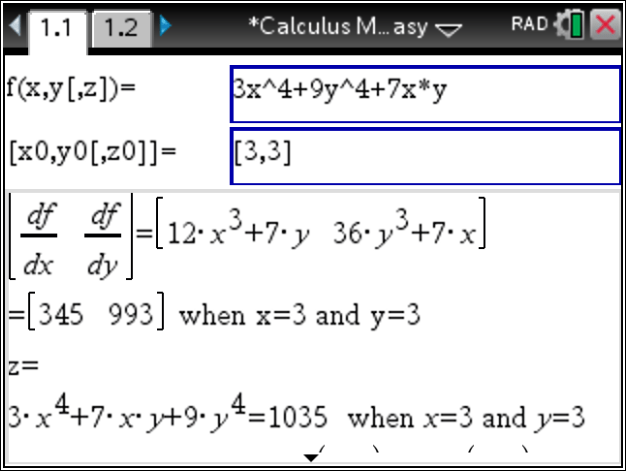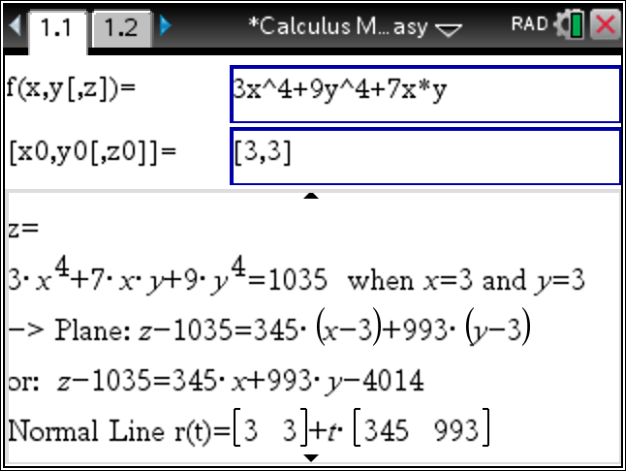## Tangent Plane – Step by Step – using the TiNspire CX

QUESTION:  Find an equation of the tangent plane to the surface
z=3x^4+9y^4+7xy at the point (3,3,1035).

SOLUTION: Start Calculus Made Easy , go to the Multivariable Calculus in the menu.

There, enter as shown below :The steps are shown in the box below: partial derivatives are computed and evaluated. And the function is evaluated at the given point . Thus, the Tangent Plane is derived. Additionally, a line normal to the plane and a normal vector are found.Thus, finally
Posted on Categories calculus